## Example Questions

← Previous 1

### Example Question #1 : How To Find The Percent Of Increase

The population of Town A is 12,979 people in 1995.  The population, when measured again in 2005, is 22,752.  What was the change in population to the nearest whole percentage point?

68%

43%

57%

75%

175%

75%

Explanation:

Since we are looking for the change, we must take the

(Ending Point – Starting Point)/Starting Point * 100%

(22752 – 12979)/12979 * 100%

9773/12979 * 100%

0.753 * 100%

75%

### Example Question #1 : How To Find The Percent Of Increase

A factory produced 2500 units during the month of September.  In order to increase production by 12% in the month of October, the factory hired more workers.  How many units were produced in October?

2800

4000

3600

3200

3000

2800

Explanation:

This is a percentage increase problem.

Easiest approach :  2500 x 1.12 = 2800

In this way you are adding 12% to the original.

Using the formula, find 12% of 2500

12/100 = x/2500,

30000 = 100x

300 = x

Now add that to the original to find the new production:

2500 + 300 = 2800

### Example Question #3 : Percent Of Change

The radius of a given circle is increased by 20%.  What is the percent increase of the area of the circle.

100%

144%

44%

40%

20%

44%

Explanation:

If we plug-in a radius of 5, then a 20% increase would give us a new radius of 6 (which is 1.2 x 5).  The area of the new circle is π(6)2 = 36π, and the area of the original circle was π(5)2 = 25π .  The numerical increase (or difference) is 36π - 25π = 11π.  Next we have to divide this difference by the original area: 11π/25π = .44, which multiplied by 100 gives us a percent increase of 44%.  The percent increase = (the numerical increase between the new and original values)/(original value) x 100. The algebraic solution gives us the same answer.  If radius r of a certain circle is increased by 20%, then the new radius would be (1.2)r.  The area of the new circle would be 1.44 π r2 and the area of the original circle πr2.  The difference between the areas is .44 π r2, which divided by the original area, π r2, would give us a percent increase of .44 x 100 = 44%.

### Example Question #2 : How To Find The Percent Of Increase

Phoenicia is a grocery store that is expanding quickly.

In 2011 Phoenicia's total sales were $1,800,800. In 2012 their sales rose to$2,130,346.

By what percentage did the store increase its income from 2011 to 2012.

(Round answer to the nearest tenth.)

10.5%

21.0%

16.4%

19.2%

18.3%

18.3%

Explanation:

$1,800,800 divided by 100 equals 18,008 and$2,130,346 divided by 18,008 is 118.3

So we know that $2,130,346 is 118.3% of the sales in the previous year. Hence sales increased by 18.3%. ### Example Question #1 : How To Find The Percent Of Increase Cindy is running for student body president and is making circular pins for her campaign. She enlarges her campaign image to fit the entire surface of a circular pin. After the image is enlarged, its new diameter is 75 percent larger than the original. By approximately what percentage has the area of the image increased? Possible Answers: 20% 225% 75% 100% 200% Correct answer: 200% Explanation: Pick any number to be the original diameter. 10 is easy to work with. If the diameter is 10, the radius is 5. The area of the original image is A = πr2, so the original area = 25π. Now we increase the diameter by 75%, so the new diameter is 17.5. The radius is then 8.75. The area of the enlarged image is approximately 77π. To find the percentage by which the area has increased, take the difference in areas divided by the original area. (77π - 25π)/25π = 51π/25π = 51/25 = 2.04 or approximately 200% ### Example Question #1 : How To Find The Percent Of Increase If the side of a square is doubled in length, what is the percentage increase in area? Possible Answers: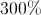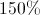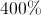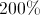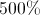Correct answer:Explanation: The area of a square is given by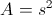, and if the side is doubled, the new area becomes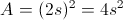. The increase is a factor of 4, which is 400%. ### Example Question #1 : How To Find The Percent Of Increase A stock for YUM was trading at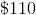. If the price increased by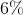, then decreased by, then increased by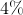; what was the net % change in price (to the nearest tenth of a percent)? (use a negative sign to denote a decrease) Possible Answers: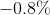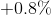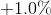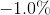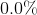Correct answer:Explanation: We will use the formula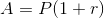to solve this one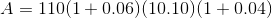compute the terms in the parentheses: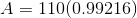If we rewrite the term in parentheses to match the form of the original formula, we can find the rate without having to do extra computation.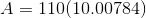So, the rate is a decrease by 0.784%, which we round to 0.8% ### Example Question #1 : How To Find The Percent Of Increase A circle has its radius increased by. By what percent is its area increased? Possible Answers: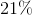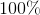Cannot be determined with the information given.Correct answer:Explanation: If we use r to denote the original radius of the circle, then according to the formula: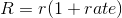the new radius R, is given by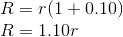Therefore, the new area is: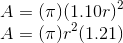Or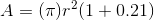Since (pi)r2 is the area of the original circle, the rate of the increase is 21% ### Example Question #1 : How To Find The Percent Of Decrease At a certain store, prices for all items were assigned in January. Each month after that, the price was 20% less than the price the previous month. If the price of an item was x dollars in January, approximately what was the price in dollars of the item in June? Possible Answers: 0.12x 0.81x 0.51x 0.66x 0.33x Correct answer: 0.33x Explanation: The question tells us that the price in January was x. To find the price in February, we decrease the price of by 20%, which is the same as taking 80% of x. (In general, a P% decrease of a number is the same as (100 – P)% times that number). Continue to take 0.8 times the previous month's price to find the next month's price until we have the price for June, as follows: January Price: x February Price: 0.8 * January Price = 0.8x March Price: 0.8 * February Price = 0.8 * 0.8x = 0.82 * x April Price: 0.8 * March Price = 0.8 * 0.8 * 0.8x = 0.8* x May Price: 0.8 * April Price = 0.8 * 0.8 * 0.8 * 0.8x = 0.8* x June Price: 0.8 * May Price = 0.8 * 0.8 * 0.8 * 0.8 * 0.8= 0.8* x; therefore, the price in June was 0.8≈ 0.328 ≈ 0.33 times the original price. ### Example Question #1 : How To Find The Percent Of Decrease The sale of a tablet decreased from$500 to $450. By what percentage did the cost decrease? Possible Answers: 10% 5% 1% 20% 15% Correct answer: 10% Explanation: Set up the following ratio 50/500 = n/100 The cost of the tablet decreased by$50. The original cost is \$500; therefore, 50 is the numerator and 500 is the denominator on the left side of the ratio.

Since a percentage is a part of a whole, n symbolizes the the percentage decrease.

To solve for n, you can cross multiply. So, 50(100) = n(500).

n = 10%

← Previous 1

### All SAT Math Resources